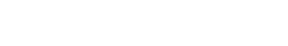# Hotelling’s T-Squared: Simple Definition

Hotelling’s T-Squared (Hotelling, 1931) is the multivariate counterpart of the T-test. “Multivariate” means that you have data for more than one parameter for each sample. For example, let’s say you wanted to compare how well two different sets of students performed in school. You could compare univariate data (e.g. mean test scores) with a t-test. Or, you could use Hotelling’s T-squared to compare multivariate data (e.g. the mutivariate mean of test scores, GPA, and class grades).

Hotelling’s T-Squared is based on Hotelling’s T2 distribution and forms the basis for various multivariate control charts.

## Test Versions

Two versions of the test exist with the following null hypotheses:

• One sample: The multivariate vector means for a group equals a hypothetical vector of means.
• Two sample: The multivariate vector of means for two groups are equal.

For more than two samples, one option is to run a MANOVA.

## Two-sample Hotelling’s T-Squared

If you know how to run a two sample t-test, then you know how to run a two-sample Hotelling’s T-squared. The basic steps are the same, although you’ll use a different formula to calculate the t-squared value and you’ll use a different table (the F-table) to find the critical value.

Hotelling’s T-squared has several advantages over the t-test (Fang, 2017):

• The Type I error rate is well controlled,
• The relationship between multiple variables is taken into account,
• It can generate an overall conclusion even if multiple (single) t-tests are inconsistent. While a t-test will tell you which variable differ between groups, Hotelling’s summarizes the between-group differences.

The test hypotheses are:

• Null hypothesis (H0): the two samples are from populations with the same multivariate mean.
• Alternate hypothesis (H1): the two samples are from populations with different multivariate means.

Three major assumptions are that the samples:Hotelling’s-T can be transformed to an F-statistic.Like the t-test, you’ll want to find a value for T (in this case, for T-squared) and compare it to a table value; if the calculated value is greater than the table statistic, you can reject the null hypothesis. For ease of this calculation, Hotelling’s t2 is first transformed into an F-statistic:Where:

• N1 & N2 = sample sizes,
• p = number of variables measured,
• N1 + N2 – p – 1 = degrees of freedom.

Reject the null hypothesis (at a chosen significance level) if the calculated value is greater than the F-table critical value. Rejecting the null hypothesis means that at least one of the parameters, or a combination of one or more parameters working together, is significantly different.

References:
Fang, J. (2017). Handbook of Medical Statistics.
Hotelling H (1931) The generalization of Student’s ratio. Ann Math Stat. 2(3):360–378.

CITE THIS AS:
Stephanie Glen. "Hotelling’s T-Squared: Simple Definition" From StatisticsHowTo.com: Elementary Statistics for the rest of us! https://www.statisticshowto.com/hotellings-t-squared/
---------------------------------------------------------------------------Need help with a homework or test question? With Chegg Study, you can get step-by-step solutions to your questions from an expert in the field. Your first 30 minutes with a Chegg tutor is free!